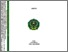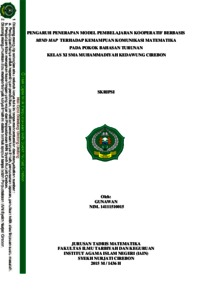# PENGARUH PENERAPAN MODEL PEMBELAJARAN KOOPERATIF BERBASIS MIND MAP TERHADAP KEMAMPUAN KOMUNIKASI MATEMATIKA PADA POKOK BAHASAN TURUNAN

GUNAWAN, (2015) PENGARUH PENERAPAN MODEL PEMBELAJARAN KOOPERATIF BERBASIS MIND MAP TERHADAP KEMAMPUAN KOMUNIKASI MATEMATIKA PADA POKOK BAHASAN TURUNAN. Bachelor thesis, IAIN Syekh Nurjati Cirebon.Preview
Text
GUNAWAN 14111510015 (WM BLM).pdf

Download (7MB) | Preview

## Abstract

INFLUENCE OF APPLICATION MODEL COOPERATIVE LEARNING BASED MIND MAP ON MATHEMATICAL COMMUNICATION SKILLS ON THE SUBJECTS OF DERIVATIVES IN CLASS XI MUHAMMADIYAH KEDAWUNG CIREBON Based on the observations, according to the teachers of mathematics courses is concerned, mathematical communication skills are still relatively low. It is seen from the difficulty of students to convey mathematical ideas in the form of mathematical symbols, still can not declare a daily occurrence in the language of mathematical symbols and there is a mathematics teacher who is focused only teaches mathematics as much as possible to students, so that students are difficult to understand. Given the importance of communication skills of mathematics, so it is developing a teaching model that is closely connected with mathematical communication skills, among others, cooperative learning model based mind map. Cooperative learning model based mind map is a way used by teachers in a series of learning activities where students are formed in specific groups then students describe and communicate the way of thinking is structured in accordance with the student's mind. So it is assumed when applied cooperative learning model based mind map then able to improve mathematical communication skills in students. The purposes of this study were to: (1) Describe the students' response to cooperative learning model based mind map, (2) Describe the mathematical communication skills by applying cooperative learning model based mind map, (3) to analyze the effect of the application of cooperative learning model based on the mind map mathematical communication skills on the subject of derivatives. Data collection techniques used in this study were questionnaires and tests. The population is all students of class XI SMA Muhammadiyah kedawung Cirebon. The sample used was a class XI IPA 1 with a sampling technique using cluster random sampling. The data analysis technique processed by using SPSS version 20 for Windows. From the analysis of data obtained by the positive response to the use of cooperative learning model based mind map by 78.41% and the average value of mathematics communication ability of students is about 77.11. After testing the hypothesis obtained that thitung (3287)> t table (1,689), then Ho is rejected and Ha accepted, meaning that there are effects of the application of cooperative learning model based mind map on mathematical communication skills. The regression equation Y = 14 216 + 0802 X. regression coefficient of 0.802 states that each additional (increased) the use of cooperative learning model based mind map will affect the communication skills of mathematics at 0.802. Coefficient is positive, it means there is a positive relationship between cooperative learning model based mind map on mathematical communication skills. From the correlation coefficient of 0, 486 obtained by the index of determination of 0.236, which means 23.6% mathematics communication ability is determined by the use of cooperative learning model based mind map and the remaining 76.4% is influenced by other factors.

Item Type: Thesis (Bachelor) Pendidikan > Pendidikan (Umum) > Model Pembelajaran Fakultas Ilmu Tarbiyah dan Keguruan > 4. Tadris Matematika H. Tohirin S.Ag 05 Jun 2017 07:24 05 Jun 2017 07:24 http://repository.syekhnurjati.ac.id/id/eprint/2966

### Actions (login required)View Item